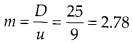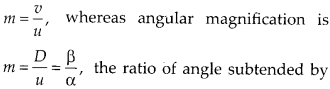Enlightened

# Question 29: NCERT Solutions for 12th Class Physics: Chapter 9-Ray Optics and Optical Instruments

• 0

Question 29: NCERT Solutions for 12th Class Physics: Chapter 9-Ray Optics and Optical Instruments

A card sheet divided into squares each of size 1 mm2 is being viewed at a distance of 9 cm through a magnifying glass (a converging lens of focal length 10 cm) held close to the eye.
(a) What is the magnification produced by the lens? How much is the area of each square in the virtual image?
(b) What is the angular magnification (magnifying power) of the lens?
(c) Is the magnification in (a) equal to the magnifying power in (b)? Explain.

Share

1. Solution:
(a) For magnification by the magnifying lens.(b) Angular magnification,(c) No, the linear magnification by a lens and magnifying power (angular magnification) of magnifying glass have different values. The linear magnification is calculated usingimage of object at eye lens ‘p’ to the angle subtended by object assumed to be at least distance at eye lens ‘a’.
The linear magnification and angular magnification in microscope have similar magnitude when image is at least distance of distinct vision i.e., 25 cm.

Check the complete chapter with solutions.

NCERT Solutions for 12th Class Physics: Chapter 9-Ray Optics and Optical Instruments

• 0